# microproject@math (1).pdf

25 Apr 2023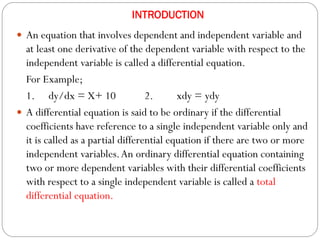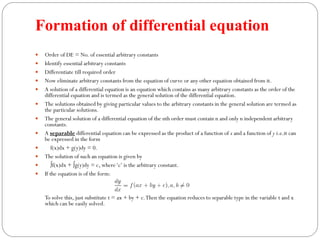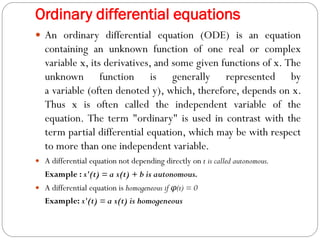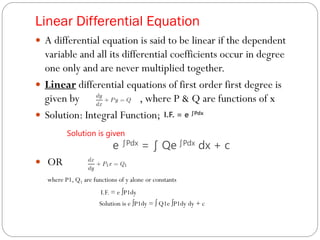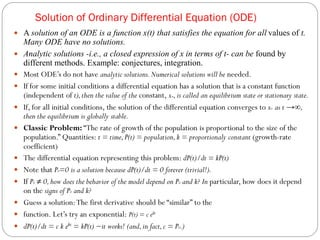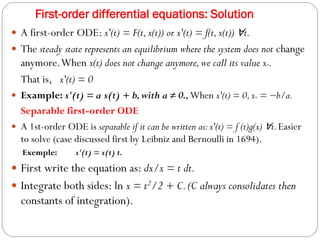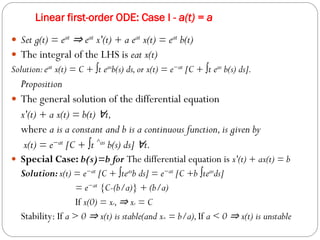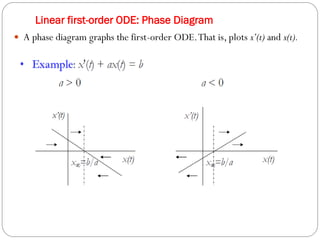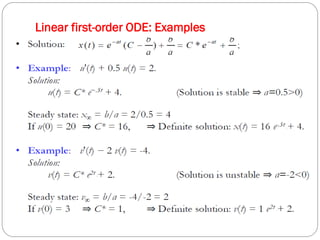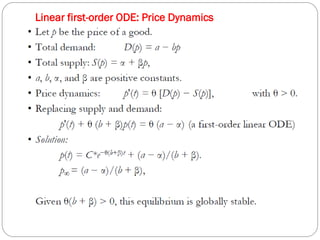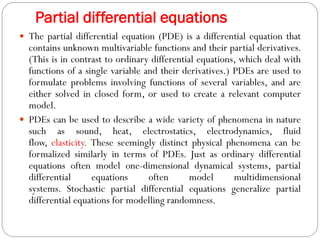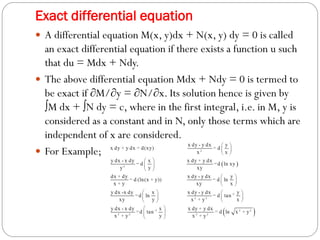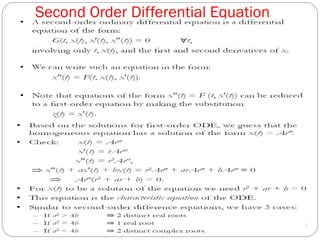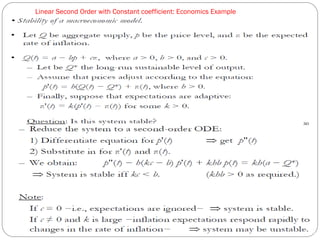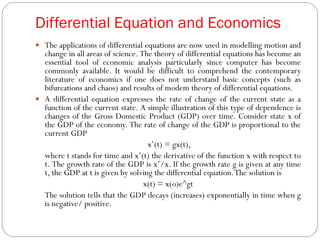1 sur 16

### microproject@math (1).pdf

• 2. INTRODUCTION  An equation that involves dependent and independent variable and at least one derivative of the dependent variable with respect to the independent variable is called a differential equation. For Example; 1. dy/dx = X+ 10 2. xdy = ydy  A differential equation is said to be ordinary if the differential coefficients have reference to a single independent variable only and it is called as a partial differential equation if there are two or more independent variables.An ordinary differential equation containing two or more dependent variables with their differential coefficients with respect to a single independent variable is called a total differential equation.
• 3. Formation of differential equation  Order of DE = No. of essential arbitrary constants  Identify essential arbitrary constants  Differentiate till required order  Now eliminate arbitrary constants from the equation of curve or any other equation obtained from it.  A solution of a differential equation is an equation which contains as many arbitrary constants as the order of the differential equation and is termed as the general solution of the differential equation.  The solutions obtained by giving particular values to the arbitrary constants in the general solution are termed as the particular solutions.  The general solution of a differential equation of the nth order must contain n and only n independent arbitrary constants.  A separable differential equation can be expressed as the product of a function of x and a function of y i.e.it can be expressed in the form  f(x)dx + g(y)dy = 0.  The solution of such an equation is given by  ∫f(x)dx + ∫g(y)dy = c, where‘c’ is the arbitrary constant.  If the equation is of the form: To solve this, just substitute t = ax + by + c.Then the equation reduces to separable type in the variable t and x which can be easily solved.
• 4. Ordinary differential equations  An ordinary differential equation (ODE) is an equation containing an unknown function of one real or complex variable x, its derivatives, and some given functions of x. The unknown function is generally represented by a variable (often denoted y), which, therefore, depends on x. Thus x is often called the independent variable of the equation. The term "ordinary" is used in contrast with the term partial differential equation, which may be with respect to more than one independent variable.  A differential equation not depending directly on t is called autonomous. Example : x'(t) = a x(t) + b is autonomous.  A differential equation is homogeneous if φ(t) = 0 Example: x'(t) = a x(t) is homogeneous
• 5. Linear Differential Equation  A differential equation is said to be linear if the dependent variable and all its differential coefficients occur in degree one only and are never multiplied together.  Linear differential equations of first order first degree is given by , where P & Q are functions of x  Solution: Integral Function; Solution is given e ∫Pdx = ∫ Qe ∫Pdx dx + c  OR where P1, Q1 are functions of y alone or constants I.F. = e ∫P1dy Solution is e ∫P1dy = ∫ Q1e ∫P1dy dy + c I.F. = e ∫Pdx
• 6. Solution of Ordinary Differential Equation (ODE)  A solution of an ODE is a function x(t) that satisfies the equation for all values of t. Many ODE have no solutions.  Analytic solutions -i.e., a closed expression of x in terms of t- can be found by different methods. Example: conjectures, integration.  Most ODE’s do not have analytic solutions.Numerical solutions will be needed.  If for some initial conditions a differential equation has a solution that is a constant function (independent of t),then the value of the constant, x∞,is called an equilibrium state or stationary state.  If, for all initial conditions, the solution of the differential equation converges to x∞ as t →∞, then the equilibrium is globally stable.  Classic Problem: “The rate of growth of the population is proportional to the size of the population.” Quantities: t = time,P(t) = population,k = proportionaly constant (growth-rate coefficient)  The differential equation representing this problem: dP(t)/dt = kP(t)  Note that P0=0 is a solution because dP(t)/dt = 0 forever (trivial!).  If P0 ≠ 0,how does the behavior of the model depend on P0 and k? In particular, how does it depend on the signs of P0 and k?  Guess a solution:The first derivative should be “similar” to the  function. Let’s try an exponential: P(t) = c ekt  dP(t)/dt = c k ekt = kP(t) −it works! (and,in fact,c = P0.)
• 7. First-order differential equations: Solution  A first-order ODE: x'(t) = F(t,x(t)) or x'(t) = f(t,x(t)) ∀t.  The steady state represents an equilibrium where the system does not change anymore.When x(t) does not change anymore,we call its value x∞. That is, x'(t) = 0  Example: x'(t) = a x(t) + b,with a ≠ 0.,When x'(t) = 0,x∞ = −b/a. Separable first-order ODE  A 1st-order ODE is separable if it can be written as:x'(t) = f (t)g(x) ∀t.Easier to solve (case discussed first by Leibniz and Bernoulli in 1694). Exemple: x'(t) = x(t) t.  First write the equation as: dx/x = t dt.  Integrate both sides: ln x = t2/2 + C.(C always consolidates then constants of integration).
• 8. Linear first-order ODE: Case I - a(t) = a  Set g(t) = eat ⇒ eat x'(t) + a eat x(t) = eat b(t)  The integral of the LHS is eat x(t) Solution:eat x(t) = C + ∫t easb(s) ds,or x(t) = e−at [C + ∫t eas b(s) ds]. Proposition  The general solution of the differential equation x'(t) + a x(t) = b(t) ∀t, where a is a constant and b is a continuous function,is given by x(t) = e−at [C + ∫t ^as b(s) ds] ∀t.  Special Case: b(s)=b for The differential equation is x'(t) + ax(t) = b Solution:x(t) = e−at [C + ∫teasb ds] = e−at [C +b ∫teasds] = e−at {C-(b/a)} + (b/a) If x(0) = x0,⇒ x0 = C Stability: If a > 0 ⇒ x(t) is stable(and x∞ = b/a),If a < 0 ⇒ x(t) is unstable
• 9. Linear first-order ODE: Phase Diagram  A phase diagram graphs the first-order ODE.That is, plots x’(t) and x(t).
• 10. Linear first-order ODE: Examples
• 11. Linear first-order ODE: Price Dynamics
• 12. Partial differential equations  The partial differential equation (PDE) is a differential equation that contains unknown multivariable functions and their partial derivatives. (This is in contrast to ordinary differential equations, which deal with functions of a single variable and their derivatives.) PDEs are used to formulate problems involving functions of several variables, and are either solved in closed form, or used to create a relevant computer model.  PDEs can be used to describe a wide variety of phenomena in nature such as sound, heat, electrostatics, electrodynamics, fluid flow, elasticity. These seemingly distinct physical phenomena can be formalized similarly in terms of PDEs. Just as ordinary differential equations often model one-dimensional dynamical systems, partial differential equations often model multidimensional systems. Stochastic partial differential equations generalize partial differential equations for modelling randomness.
• 13. Exact differential equation  A differential equation M(x, y)dx + N(x, y) dy = 0 is called an exact differential equation if there exists a function u such that du = Mdx + Ndy.  The above differential equation Mdx + Ndy = 0 is termed to be exact if ∂M/∂y = ∂N/∂x. Its solution hence is given by ∫M dx + ∫N dy = c, where in the first integral, i.e. in M, y is considered as a constant and in N, only those terms which are independent of x are considered.  For Example;
• 14. Second Order Differential Equation
• 15. Linear Second Order with Constant coefficient: Economics Example
• 16. Differential Equation and Economics  The applications of differential equations are now used in modelling motion and change in all areas of science. The theory of differential equations has become an essential tool of economic analysis particularly since computer has become commonly available. It would be difficult to comprehend the contemporary literature of economics if one does not understand basic concepts (such as bifurcations and chaos) and results of modem theory of differential equations.  A differential equation expresses the rate of change of the current state as a function of the current state. A simple illustration of this type of dependence is changes of the Gross Domestic Product (GDP) over time. Consider state x of the GDP of the economy. The rate of change of the GDP is proportional to the current GDP x’(t) = gx(t), where t stands for time and x’(t) the derivative of the function x with respect to t. The growth rate of the GDP is x’/x. If the growth rate g is given at any time t, the GDP at t is given by solving the differential equation.The solution is x(t) = x(o)e^gt The solution tells that the GDP decays (increases) exponentially in time when g is negative/ positive.